# Stopping Distance Formula

Stopping Distance Formula

If a driver puts on the brakes of a car, the car will not come to a stop immediately. The stopping distance is the distance the car travels before it comes to a rest. It depends on the speed of the car and the coefficient of friction (μ) between the wheels and the road. This stopping distance formula does not include the effect of anti-lock brakes or brake pumping. The SI unit for stopping distance is meters.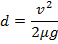d = stopping distance (m)

v = velocity of the car (m/s)

μ = coefficient of friction (unitless)

g = acceleration due to gravity (9.80 m/s2)

Stopping Distance Formula Questions:

1) A driver in a car on a residential street is traveling at 50.0 km/h. She puts on the brakes when she sees a stop sign. The coefficient of friction between the tires and the road is μ = 0.60. What is the stopping distance of the car?

Answer: The speed of the car must be converted to meters per second: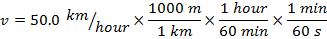v = 13.89 m/s

The stopping distance can be found using the formula: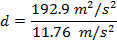d = 16.40 m

The stopping distance of the car is 16.40 m.

2) A driver in a car on an icy highway is traveling at 100.0 km/h. He puts on the brakes and begins to slide. The coefficient of friction between the tires and the ice on the road is μ = 0.15. What is the stopping distance of the car?

Answer: The speed of the car must be converted to meters per second: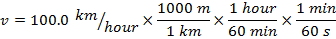v = 27.78 m/s

The stopping distance can be found using the formula: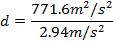d = 262.4 m

The stopping distance of the car on an icy highway is 262.4 m.